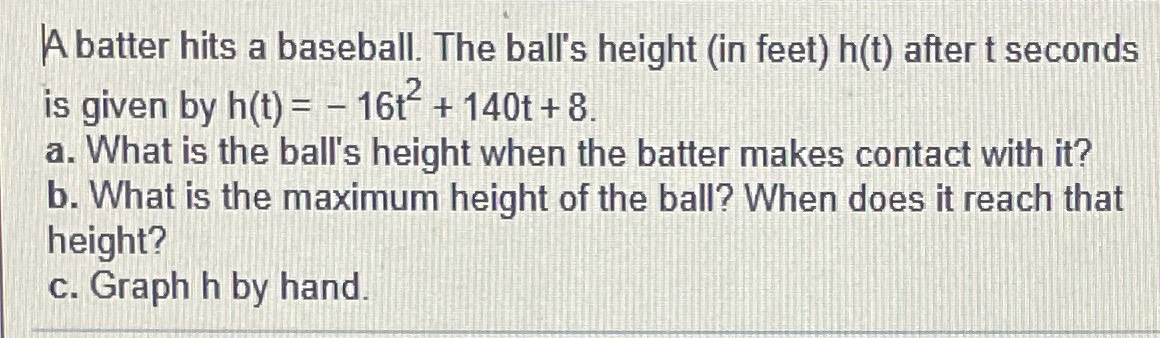### ¿Todavía tienes preguntas de matemáticas?

Pregunte a nuestros tutores expertos
Algebra
PreguntaA batter hits a baseball. The ball's height (in feet) $$h ( t )$$ after t seconds is given by $$h ( t ) = - 16 t ^ { 2 } + 140 t + 8$$ . a. What is the ball's height when the batter makes contact with it? b. What is the maximum height of the ball? When does it reach that height? c. Graph $$h$$ by hand.